# Designing and Simulation of Industrial PID Controllers using Microcontrollers

Ing. Cristoforo Baldoni

In this article we’ll see how to pass from the design of analog PID controllers for continuous-time systems to digital controllers, replacing operational amplifiers, resistors and capacitors with microcontrollers. Digital controllers are very compact, all the controller fits on a chip, including the A/D and the D/A converters, moreover, digital controllers are not affected by the aging of the components and don’t change their values with the temperature as analog components do. We’ll see how to apply the Z-transform, the equivalent of the Laplace transform, but for discrete-time systems, we’ll see how to identify the transfer function of a process and we’ll explain, with a step by step procedure, how to apply the theoretical knowledge learnt by examining an Proteus microcontroller based project, that uses its PWM output to control an oven ‘s temperature. The microcontroller has a 10 bit A/D converter. This procedure can be easily adapted with minimal adjustments to other processes to control.

Topics

1. Digital Control-System Block Diagrams

2. Linear difference equations, Z-Transform, Inverse Z-Transform and Discrete Transfer Function.

3. Sampling and A/D Analogic to Digital Converter

4. D/A Digital to Analogic Converter and ZERO ORDER HOLD  (ZOH) : Relationship between the Continuous Transfer Function and Discrete Transfer Function of a sampled Process.

5.  Block Diagram Manipulation of Sampled Data Systems

6. Methods for designing Digital Controllers, Stability.

7. Designing  PID controllers by microcontrollers

8. Transfer Function Identification and PID Tuning using the Ziegler–Nichols Method.

9. Practical case of a temperature control system implemented with a microcontroller PIC and simulated with ISIS Proteus: Step by step explanation of how to apply the theoretical knowledge for implementing and simulating a PID controller.

# Designing Industrial PID Controllers using SPICE

1. Design of PID regulators , design and test with SPICE simulation

2. Easy way to implement compensators with operational amplifiers

1. Design of PID regulators , design and test with SPICE simulation

Graphical method

Let’s examine now a graphical method for designing P.I.D. controllers. P.I.D. are Proportional-Integral-Derivative controllers and represent one of the techniques widely used to implement industrial control systems. Below the diagram block of a typical feedback systemFigure 1 – Generic feedback system

There are several types of compensators, in series, in feedback and combinations of both. The compensator in series is the most used. A compensation system is required when the closed loop system is unstable, when the system is stable but the steady state error or the dynamic behavior doesn’t meet the required specifications, or both. To compensate the system we put before the process (in series) a controller whose transfer function is R(s), as you can see in the figure belowFigure 2 – Compensated feedback system

The generic transfer function introduced by the P.I.D. controller can be expressed as:(0.1)

P.I.D. controllers can exist also as a simple P controller (KD = KI =0), as a P.D. controller (KI = 0), or as a P.I. controller (KD =0). The proportional contribute of a P controller can improve the specification about the steady state error, the derivative contribute increases the damping of the closed loop system and the integrative contribute increases the type of the system, and then can eliminate or improve the steady state error.

P CONTROLLER.

The simplest version is the proportional controller P. Its transfer function is:(0.2)

This type of controller can improve the specification on the steady state error, but at the same time increases the bandwidth of the feedback system which means a faster response of the system to follow the reference but a decrease of the stability margins.  For a system with unitary feedback and open loop transfer function equal to F(s) the steady state error is: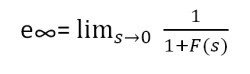(0.3)

multiplying by the gain Kp the new error will be(0.4)

let’s see a practical example, the open loop transfer function of a unitary feedback system is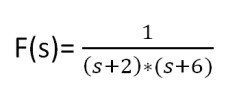(0.5)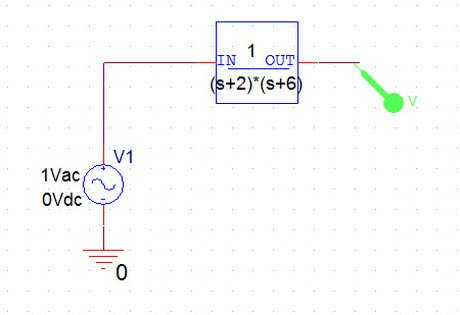Figure 3 – Implementation of open loop function with Laplace PART in PSpice

The bode plot of the transfer function is:Figure 4 – Bode plot of gain and phase

The specification on the system requires, for example, a steady state error equal to 3% and a phase margin PM of 50 degrees.It’s: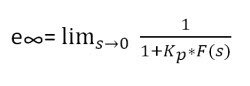(0.6)

we have to impose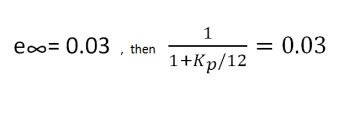(0.7)

we get KP= 388.

Plotting the compensated function we getFigure 5 – Bode plot of function compensated with proportional controller

The phase corresponding to the crossing frequency is about -156 degrees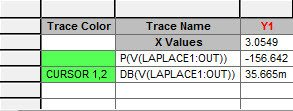Figure 6 – Phase at crossing frequency

the specific on the phase margin can’t be satisfied with only the proportional compensation.